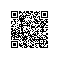# 棋盘覆盖问题的算法实现

在一个2^k * 2^k个方格组成的棋盘中，有一个方格与其它的不同，若使用以下四种L型骨牌覆盖除这个特殊方格的其它方格，如何覆盖。

四各L型骨牌如下图1图1实现的基本原理是将2^k * 2^k的棋盘分成四块2^(k - 1) * 2^(k - 1)的子棋盘，特殊方格一定在其中的一个子棋盘中，如果特殊方格在某一个子棋盘中，继续递归处理这个子棋盘，直到这个子棋盘中只有一个方格为止如果特殊方 格不在某一个子棋盘中，将这个子棋盘中的相应的位置设为骨牌号，将这个无特殊方格的了棋盘转换为有特殊方格的子棋盘，然后再递归处理这个子棋盘。以上原理 如图3所示。将棋盘保存在一个二维数组中。骨牌号从1开始，特殊方格为0，如果是一个4 * 4的棋盘，特殊方格为(2,2)，那么程序的输出为

2   2   3   3
2   1   1   3
4   1   0   5
4   4   5   5

#include <stdio.h>

#define BOARD_SIZE 4
int board[BOARD_SIZE][BOARD_SIZE];

// c1, r1: 棋盘左上角的行号和列号
// c2, r2: 特殊方格的行号和列号
// size = 2 ^ k
void chessboard(int r1, int c1, int r2, int c2, int size)
{

if(1 == size) return;

int half_size;

static int domino_num = 1;

int d = domino_num++;
half_size
= size / 2;

if(r2 < r1 + half_size && c2 < c1 + half_size) //特殊方格在左上角子棋盘
{
chessboard(r1, c1, r2, c2, half_size);
}

else   // 不在此棋盘，将此棋盘右下角设为相应的骨牌号
{
board[r1
+ half_size - 1][c1 + half_size - 1= d;
chessboard(r1, c1, r1
+ half_size - 1, c1 + half_size - 1, half_size);
}

if(r2 < r1 + half_size && c2 >= c1 + half_size) //特殊方格在右上角子棋盘
{
chessboard(r1, c1
+ half_size, r2, c2, half_size);
}

else  // 不在此棋盘，将此棋盘左下角设为相应的骨牌号
{
board[r1
+ half_size - 1][c1 + half_size] = d;
chessboard(r1, c1
+ half_size, r1 + half_size - 1, c1 + half_size, half_size);
}

if(r2 >= r1 + half_size && c2 < c1 + half_size) //特殊方格在左下角子棋盘
{
chessboard(r1
+ half_size, c1, r2, c2, half_size);
}

else  // 不在此棋盘，将此棋盘右上角设为相应的骨牌号
{
board[r1
+ half_size][c1 + half_size - 1= d;
chessboard(r1
+ half_size, c1, r1 + half_size, c1 + half_size - 1, half_size);
}

if(r2 >= r1 + half_size && c2 >= c1 + half_size) //特殊方格在左上角子棋盘
{
chessboard(r1
+ half_size, c1 + half_size, r2, c2, half_size);
}

else   // 不在此棋盘，将此棋盘左上角设为相应的骨牌号
{
board[r1
+ half_size][c1 + half_size] = d;
chessboard(r1
+ half_size, c1 + half_size, r1 + half_size, c1 + half_size, half_size);
}
}

int main()
{

int i, j;
board[
2][2= 0;
chessboard(
0022, BOARD_SIZE);

for(i = 0; i < BOARD_SIZE; i++)
{

for(j = 0; j < BOARD_SIZE; j++)
{
printf(
"%-4d", board[i][j]);
}
printf(
"/n");
}
}

《Java Web开发速学宝典》出版，欢迎定购使用钉钉扫一扫加入圈子
+ 订阅